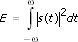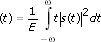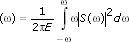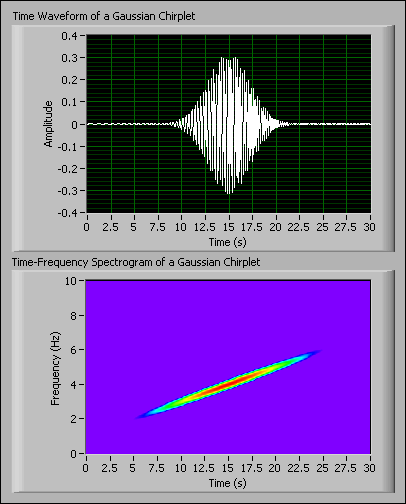# Central Time and Central Frequency (Advanced Signal Processing Toolkit)

LabVIEW 2014 Advanced Signal Processing Toolkit Help

Edition Date: June 2014

Part Number: 372656C-01

»View Product InfoDownload Help (Windows Only)Note  This topic contains information that applies to the LabVIEW Time Frequency Analysis Tools.

You can express a signal as a linear combination of a series of elementary functions. For example, the Gabor transform decomposes a signal into a linear combination of Gabor elementary functions, which are determined by dual windows. Similarly, the adaptive transform decomposes a signal as a linear combination of Gaussian chirplet or Gaussian pulse elementary functions, which are calculated by the matching pursuit method.

Each elementary function locates a different position in the time and frequency domains of a signal. In the time domain, the position is the central time. In the frequency domain, the position is the central frequency.

The following equations define the central time and the central frequency of a signal, s(t).where E is the energy, or power, of the signal t is time (t) is the central time of the signal () is the central frequency of the signal S() is the Fourier transform of the signal

Use the LabVIEW Time Frequency Analysis Tools to decompose a signal as a linear combination of elementary functions. You can observe the central time and central frequency by plotting the time waveform and the time-frequency spectrogram of the signal, respectively.

The following illustrations show the time waveform and the time-frequency spectrogram of a Gaussian chirplet. You can see that the central time of this chirplet is 15 s and the central frequency of this chirplet is 4 Hz.